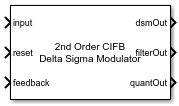# Delta Sigma Modulator

Model a discrete delta sigma modulator ADC

• Library:
• Mixed-Signal Blockset / ADC / Building Blocks

•## Description

Use the Delta Sigma Modulator block to model a discrete delta sigma modulator based ADC using a set of different architectures such as cascade of feedback or feed-forward resonators or integrators.​ You can model an ADC of orders two to six. You can model circuit based noise and also get accurate switched capacitor values used in actual circuit design and layout.

## Ports

### Input

expand all

Data Types: `double`

Reset signal for Delta Sigma Modulator block, specified as a scalar.

Data Types: `double`

### Output

expand all

Digital output signal, returned as a scalar.

Data Types: `fixed point` | `single` | `double` | `int8` | `int16` | `int32` | `uint8` | `uint16` | `uint32` | `Boolean`

## Parameters

expand all

Architecture of the delta sigma modulator, specified as:

• `CIFB` — cascade of feedback integrators.

• `CRFB` — cascade of feedback resonators.

• `CIFF` — cascade of feed-forward integrators.

• `CRFF` — cascade of feed-forward resonators.

#### Programmatic Use

 Block parameter: `dsmArchitecture` Type: character vector Values: `CIFB` | `CRFB` | `CIFF` | `CRFF` Default: `CIFB`

Order of delta sigma modulator. You can choose between orders two to six.

#### Programmatic Use

 Block parameter: `dsmOrder` Type: character vector Values: `2nd order` | `3rd order` | `4th order` | `5th order` | `6th order` Default: `2nd order`
Input Parameters

Sampling frequency of the delta sigma modulator, specified as a positive real scalar in Hz.

#### Programmatic Use

 Block parameter: `SamplingFrequency` Type: character vector Values: positive real scalar Default: `50000`

Number of quantizer levels in delta sigma modulator, specified as a positive real scalar.

#### Programmatic Use

 Block parameter: `NumberLevels` Type: character vector Values: positive real scalar Default: `2`

Range of the quantizer output in the delta sigma modulator architecture, specified as a vector with real elements.

#### Programmatic Use

 Block parameter: `QuantizerOutput` Type: character vector Values: real valued vector Default: `[-1;1]`

Feedback coefficients from the quantizer or feed-forward coefficients to the quantizer, specified as a vector with real elements.

#### Programmatic Use

 Block parameter: `a` Type: character vector Values: real valued vector Default: `[0.26017;0.2207]`

Resonator coefficients, specified as a vector with real elements.

#### Programmatic Use

 Block parameter: `g` Type: character vector Values: real valued vector Default: `[0.014544]`

Feed-in coefficients from the modulator input to each integrator, specified as a vector with real elements.

#### Programmatic Use

 Block parameter: `b` Type: character vector Values: real valued vector Default: `[0.26017;0;0]`

Integrator inter-stage coefficients, specified as a vector with real elements.

#### Programmatic Use

 Block parameter: `c` Type: character vector Values: real valued vector Default: `[0.36129;6.8191]`
Noise

Enable noise calculations in the delta sigma modulator ADC.

Ratio of signal power to noise power, specified as a nonnegative real scalar in dB.

#### Programmatic Use

 Block parameter: `SNR` Type: character vector Values: nonnegative real scalar Default: `75`

Power of the signal at the input of the delta sigma modulator, specified as a real scalar in watts.

#### Programmatic Use

 Block parameter: `InputPower` Type: character vector Values: real scalar Default: `0.125`

Bandwidth of the delta sigma modulator system,

#### Programmatic Use

 Block parameter: `BandWidth` Type: character vector Values: nonnegative real scalar Default: `1000`
Switch Capacitors

Click to turn on the calculation of switched capacitance.

Ratio of the delta sigma modulator sampling rate to the Nyquist rate, defined as a nonnegative real scalar. A high oversampling ratio results in a relaxed anti-aliasing filter and reduced in-band quantization noise.

#### Programmatic Use

 Block parameter: `OSR` Type: character vector Values: nonnegative real scalar Default: `25`

Target SNR of the delta sigma modulator system, defined as a nonnegative real scalar in dB.

#### Programmatic Use

 Block parameter: `capacitorSNR` Type: character vector Values: nonnegative real scalar Default: `75`

Supply voltage value of the CMOS technology used to design the delta sigma modulator, specified as a nonnegative real scalar. The Supply Voltage (V) is defined in the PDK.

#### Programmatic Use

 Block parameter: `SupplyRail` Type: character vector Values: nonnegative real scalar Default:

Estimated input signal swing in the CMOS technology used to design the delta sigma modulator, specified as a nonnegative real scalar in voltage. The Input Signal Swing (V) is defined in the PDK.

#### Programmatic Use

 Block parameter: `VInput` Type: character vector Values: nonnegative real scalar Default: `0.2`

Typical reference voltage value for the CMOS technology used to design the delta sigma modulator, specified as a nonnegative real scalar in voltage. The Reference Voltage (V) is defined in the PDK.

#### Programmatic Use

 Block parameter: `VReference` Type: character vector Values: nonnegative real scalar Default: `0.3`

Capacitor density of the CMOS technology used to design the delta sigma modulator, specified as a nonnegative real scalar in ff/µm. The Capacitor Density (ff/um) is defined in the PDK.

#### Programmatic Use

 Block parameter: `CapacitorDensity` Type: character vector Values: nonnegative real scalar Default: `1.5`

Fringe capacitance of the CMOS technology used to design the delta sigma modulator, specified as a nonnegative real scalar in ff/µm. The Fringe Capacitance (ff/um) is defined in the PDK.

#### Programmatic Use

 Block parameter: `FringeCapacitor` Type: character vector Values: nonnegative real scalar Default: `0.2`

Capacitor temperature coefficient in the CMOS technology used to design the delta sigma modulator, specified as a nonnegative real scalar in ppm/℃. The Capacitor Temperature Coefficient (ppm/deg-C) is defined in the PDK.

#### Programmatic Use

 Block parameter: `CapacitorCoefficient` Type: character vector Values: nonnegative real scalar Default: `2.2`

Minimum grid step allowed for designing the capacitor layout, specified as a nonnegative real scalar in µm

#### Programmatic Use

 Block parameter: `GridStep` Type: character vector Values: nonnegative real scalar Default: `0.005`

Click to calculate the switched capacitance.

## Version History

Introduced in R2021b